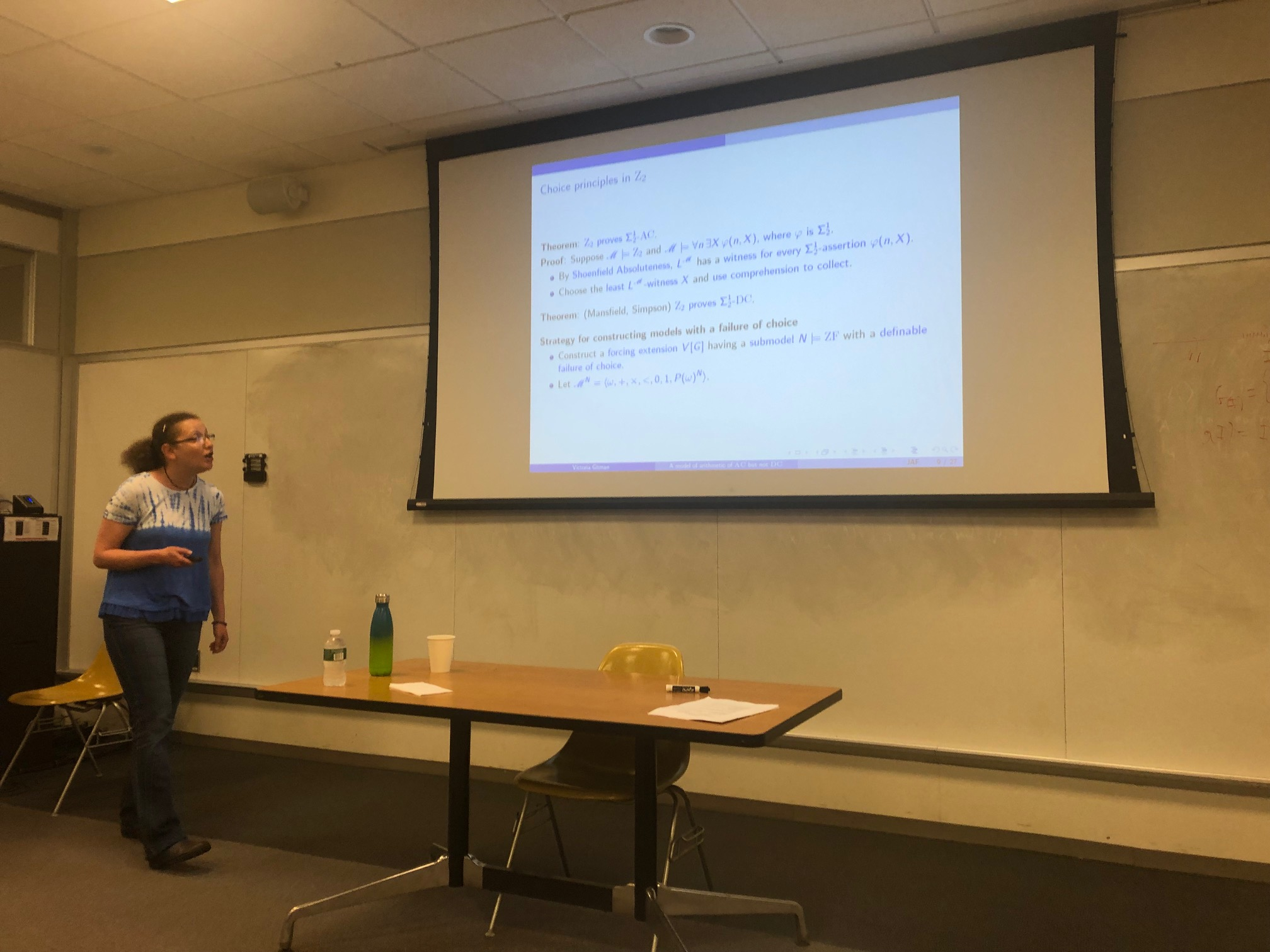# A model of second-order arithmetic satisfying ${\rm AC}$ but not ${\rm DC}$

This is a talk at the Journées sur les Arithmétiques Faibles 2019 Conference, CUNY Graduate Center, May 28-30, 2019.
Slides

Abstract: Models of second-order arithmetic have two kinds of objects: numbers and sets of numbers, which we think of as the reals. A second-order arithmetic axiom system specifies general existence rules for real numbers. If a result from, say, analysis can be proved in some such system, then we have a bound on the kind of reals that must exist in order for it to hold. Indeed, most classical results in analysis don't just follows from, but are actually equivalent (over a weak base system) to one of a short list of main second-order arithmetic systems.

One of the strongest second-order arithmetic systems is full second-order arithmetic ${\rm Z}_2$ which asserts that every second-order formula (with any number of set quantifiers) defines a set. We can augment ${\rm Z}_2$ with choice principles such as the choice scheme and the dependent choice scheme. The $\Sigma^1_n$-choice scheme asserts for every $\Sigma^1_n$-formula $\varphi(n,X)$ that if for every $n$, there is a set $X$ witnessing $\varphi(n,X)$, then there is a single set $Z$ whose $n$-th slice $Z_n$ is a witness for $\varphi(n,X)$. The $\Sigma^1_n$-dependent choice scheme asserts that every $\Sigma^1_n$-relation $\varphi(X,Y)$ without terminal nodes has an infinite branch: there is a set $Z$ such that $\varphi(Z_n,Z_{n+1})$ holds for all $n$. The system ${\rm Z}_2$ proves the $\Sigma^1_2$-choice scheme and the $\Sigma^1_2$-dependent choice scheme. The independence of $\Pi^1_2$-choice scheme from ${\rm Z}_2$ follows by taking a model of ${\rm Z}_2$ whose sets are the reals of the Feferman-Levy model of ${\rm ZF}$ in which every $\aleph_n^L$ is countable and $\aleph_\omega^L$ is the first uncountable cardinal.

We construct a model of ${\rm ZF}+{\rm AC}_\omega$ whose reals give a model of ${\rm Z}_2$ together with the full choice scheme in which $\Pi^1_2$-dependent choice fails. This result was first proved by Kanovei in 1979 and published in Russian. It was rediscovered by Sy Friedman and myself with a slightly simplified proof.